# Putting it all together – Final Project Evaluation Model [Part 6 of 6]

Share

This is a guest post written by Paramdeep from Pristine. Chandoo.org is partnering with Pristine to bring an excel financial modeling online training program for you.

###This is Part 6 of 6 on Financial Modeling using Excel

In this tutorial we are going to learn how to build assumptions & input sheets in our excel financial model. The 6 parts of this tutorial are,

I am sorry for the slight delay in the post. Things have been very hectic for the last few weeks as we were just completing our training on Financial Modeling in Excel – Real Estate (RE) sector for JP Morgan. The real estate valuation is very similar to the project evaluation that we are doing for the simple reason:

• Project evaluation and real estate valuation are limited duration projects (If you intend to sell the RE project in near future) unlike general companies (which are an on-going concern)
• For both these the timing of the cash is very important. A delay in the timing might appear to be ok for the developer, but the investor’s calculations (typically IRR) go for a toss

In India most of the RE developers are businessmen, who are concerned about the cash that the project generates. They are not really too bothered if they receive it in April or September (After all they are getting the cash). But the investors are really bothered by these delays – Some PE investors have a limited period investment horizon and some are too concerned about the IRR generated by the project. As we figured out in our class, Real estate projects are very sensitive to delays in cash generation! Excel is a great tool to show this effect in a matter of 30 secs (Use XIRR and data-tables). Maybe I will write about this functionality in one of my posts later!

For the time being lets come back to our project.

### What is time value of money?

1. If you invest \$100 in bank today, what would be its value 1 year down the line (assuming 10% interest rate)?
2. The value should be 100*(1+10%) = \$ 110.
3. Now if you keep this invested for another year, what would be its value 2 years down the line?
4. The value should be 110*(1+10%) = \$ 121. I can also write it as 110*(1+10%)^2
5. Similarly if you keep invested for 10 years, the value would be 110 * (1+10%)^10

This is the simple concept of compounding.

The inverse of this concept (What if you wanted \$110 after 1 year, or 110*(1+10%)^10 after 10 years), how much should you invest today, is called discounting. Clearly \$100 today is worth \$110 a year after and \$121 two years hence.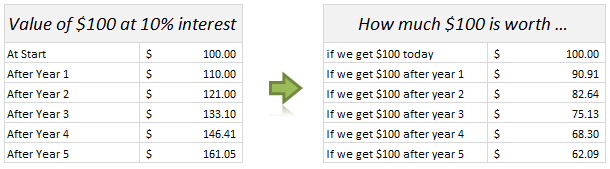If I have more than 1 cash flows, I can discount them depending on the time duration and if I sum them all, its called Net Present Value (NPV) of all cash flows. We would take the outflows as Negative Cash and inflows as Positive Cash.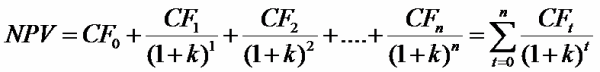In excel, you can either discount all cash flows or calculate the NPV of the project by using the function =NPV(Discount Rate, Cash)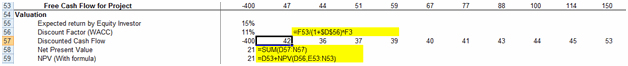### What is the rate on which money should be discounted?

When equity investors invest, they take greater risk as compared to banks lending money. Obviously their expectation of return would be higher. In some cases, the equity investor might have a return figure in mind (Based on the risk I am taking, I would like to have Min. 15% return on my invested money).

Sometimes, this expected return can be calculated by using the capital asset pricing model (CAPM). What this states is very simple – Equity investors want a premium apart from the risk free rate (Lets call this expectation of equity investors as Re) . So there are two parts to the return expectation:

Re = Risk Free + Premium apart from Risk free

Now this premium depends on how much risk I am taking (Typically measured with respect to the volatility in returns with respect to the benchmark index). So I say:

Re = Risk Free + Beta * (Market Returns – Risk Free Returns)

The beta measures the movement of your returns with respect to market returns.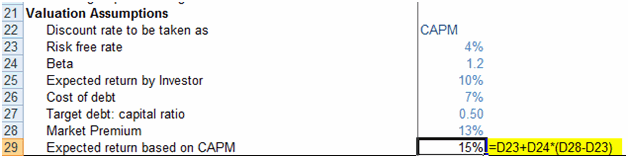Now apart from the equity investors, there would be some debt in the project. Typically debt holders expect a lower return (Lets call it Rd).

The overall expectation of return from the project is the weighted average of these returns, Re and Rd.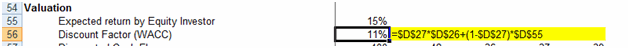To create this switch in the model, I have used data-validation (so that the user can just input one of these options)To create such a drop down, use data validation – list option in excel### Internal Rate of Return

The same concept can be viewed from a return angle as well. If I can calculate a discount rate that makes the present value of the expected cash inflows just equal to the initial cost of the project, then that rate would be sort of a break even rate for me (Considering the time value of money). This rate is called the Internal rate of return (IRR).Many investors have a certain hurdle IRR in mind and if the project is generating an IRR less than the hurdle IRR, they would not invest in the project.

To calculate IRR, there is no analytical solution possible. You can use the excel function =IRR(Cash) to get the IRR of the cash flows.

### Making a decision in our case

First let me summarize the decision criteria for you. I would invest in the project based on the following conditions:

#### NPV Rule:

• If NPV > 0: The project may be accepted (Please note that positive NPV is not a sufficient condition)
• If NPV = 0: The investor should be indifferent
• If NPV < 0: The project must not be accepted (Please note that positive NPV is a necessary condition)

Please note that sometimes people might decide to take on the project even though the NPV is negative!

#### IRR Decision Rule

• If IRR > the required rate of return, accept the project
• If IRR < the required rate of return, reject the project

In our case, we are getting the NPV to be 21 and an IRR to be 12%. In this case it’s a borderline case and my feeling is that Mr. Samar would invest in the project (After all Mohit is his son!!) J.

I have created a template for you, where the subheadings are given and you have to link the model to get the cash numbers! You can download the same from here:

Project Valuation Template – Blank

You can go through the case and fill in the yellow boxes. I also recommend that you try to create this structure on your own (so that you get a hang of what information is to be recorded).

Also you can download this filled template and check, if the information you recorded, matches mine or not!  😉

Project Valuation Template – Solution

I am just doing that for the single sheet model and recommend that you do the same for multi-sheet model as a homework problem. If you face any issue, post your excel with the exact problem and we can discuss the way to move forward.### Next Steps

We are not done with a basic model for evaluation of a project. There are other nuances that we could not tackle (Given the time and space constraint) – What if the cash does not come at year end, what could be the scenarios in which this project is not a viable project, what can be done to make the project more interesting, etc. I do hope that you found the posts interesting and look forward to your comments and suggestions!

Read previous part of this series – Modeling Cash-flow projections

### How do you make project investment decisions?

We are very eager to learn from your experience and know your ideas. What methods of valuation do you use? How do you model them? Share using comments.

Thank you Paramdeep & Pristine:

Many thanks to Paramdeep and Pristine for making this happen. I am really enjoying this series and learning a lot of valuable tricks about financial modeling.

If you like this series, say thanks to Paramdeep. I am sure he can take any amount of appreciation without choking.

Pristine is an awesome training institute for CFA, PRIMA, GARP etc. They have trained folks at HSBC, BoA etc. Chandoo.org is partnering with Pristine to bring an excel financial modeling online training program for you.

### Get FREE Excel + Power BI Tips

Simple, fun and useful emails, once per week.

Learn & be awesome.

### Welcome to Chandoo.org

Thank you so much for visiting. My aim is to make you awesome in Excel & Power BI. I do this by sharing videos, tips, examples and downloads on this website. There are more than 1,000 pages with all things Excel, Power BI, Dashboards & VBA here. Go ahead and spend few minutes to be AWESOME.

Read my storyFREE Excel tips book

I will be presenting at two events in London in April 2020. Come & join me.

Chandoo is an awesome teacher
5/5

– Jason

Still on fence about Power BI? In this getting started guide, learn what is Power BI, how to get it and how to create your first report from scratch.

### 23 Responses to “Putting it all together – Final Project Evaluation Model [Part 6 of 6]”

1.Steve Sexton says:

How would I go about modeling a proposed manufacturing project? Let's say for an example a new robotic welding cell that would cost \$300,000 and save 20% in labor.

2.Paramdeep Singh says:

Dear Steve,
The modeling steps would remain pretty much the same. Just consider only the cash flow generated because of the new project. For example,
Initial Cash Out = -30,000
Lets say Original Labor Costs (Per year) = 50,000
Savings each year = 10,000
Lets say the robot gives you benefit for 5 years. So your cash flow for the next 5 years are:
-30000 | 10000 | 10000 | 10000 | 10000 | 10000

If your WACC for the project is 15%, you can calculate the NPV of the project using NPV formula in Excel (which in this case calculates it as 3,062). If NPV is positive, it seems to be a good project to go for.

3.Steve Sexton says:

Paramdeep,

Did you miss a zero in the Initial Cash Out?

Initial Cash Out should be = -300,000.

Not -30,000.

Am I correct?

Steve

4.Paramdeep Singh says:

@steve: The initial cash has to be -30,000 and not -300,000. If you write an email to me, I will just forward you the 2 line excel sheet! 🙂

5.Shweta says:

Could you explain how is the market premium calculated?
I always understood it as:
market premium= expected return from the market- risk free rate
which in this case should be 6% (10% - 4%).
Am I missing out something?

6.Paramdeep Singh says:

@shweta: We are actually using two different approaches for valuation. In the first approach we have assumed a specific expectation by the investor (10%). In the second approach we calculate the expected return of the project using CAPM model. In the CAPM model, we have assumed a market risk premium of 13%. This can be calculated from historical data.

7.Alexkr says:

Dear Pfriends, first of all thank you very much for your efforts to educate the folks on economics! Keep moving! Meanwhile I've got two questions:
1. Am I correct the described model neglects bank loan (body) repayments? As far as I understood WACC deducts only bank interest and has nothing to do with payments on the loan itself. These repayments should significantly reduce cash flow and PAT.
2. The 'banking' part of WACC formula shall include (1 - tax) factor, but yours doesn't. Is there any mistake in your formula?

8.Richard says:

Hi Pristine and Chandoo:

Thank you for continuing to mentor us on line. I learn a lot.

Richard

9.Piyush says:

HI Paramjeet
I want to make a IRR sheet for a R& D project with certain investment. Could you pls suggest me how to make the same.
Will be thankfull for the same.
With regards
Piyush

10.Paramdeep Singh says:

Hi Piyush,
To get a model for IRR, the first step is to put the cash flows. For example, if I make an investment of USD 1000 in year 1, which gives me cash flow of 5000 growing at 10% for 5 years after 5 years, your cash flows would look like:

-1000, 0, 0, 0, 0, 5000, 5500, ...

Just use IRR function in excel after that to get the IRR for the project.
The main challenge is in getting the cash flow in place.
Hope this helps!

11. [...] months ago, we learned how to create a project valuation model in Excel as part of our series on Financial Modeling in [...]

12.Robert says:

Thank you. Excellent overview for Financial Modeling in Excel.

13.Gourav says:

You seem to ignore tax effects in the calculations. As Alexkr has pointed out, your after tax cost of debt would be 7%*(1-tax rate).

Similarly when you are calculating the cash flow benefits of Steve's new machine, you will have to take into account the fact that reduced costs (and increased profits) would mean Steve will have to shell out more money in tax. Also, we need to take into account the depreciation benefits of buying new machine. So cash flow should be (assuming 5 years life) something like:

Initial Cash Out = -30,000
Lets say Original Labor Costs (Per year) = 50,000
Savings each year = 10,000
Additional tax each year= 1200 (4,000*30%)
Steve's cash flow for the next 5 years are:
-30000 | 8,800 |8,800 |8,800 |8,800 |8,800 |
Please correct me if I am wrong.

•Steve Sexton says:

Interesting, I never really thought about the tax effect.

•Paramdeep Singh says:

Hi Gourav,
I am sorry. I did not understand the "reduced cost". I think the model is taking care of the fact that there is a depreciation (in the P&L). Also the cost of debt has the tax shield.
Please note that in the P&L the effect of interest, depreciation and tax are considered.

14.Gourav says:

Hi Paramdeep,

Apologies for being unclear. I was responding to your reply to Steve's question where you (probably inadvertently) had ignored the tax effects of cost saving. Looking at your model, I found out that it indeed taking care of the "reduced cost". The uploaded excel (where I have plugged in Steve's numbers in your template) can probably help you understand what I meant to say. The differential cash flow is coming out to be 8800 in this case.

When I am talking about after tax cost of debt, I mean this (text inspired from wiki):
Since in most cases debt expense is a deductible expense, the cost of debt is computed as an after tax cost to make it comparable with the cost of equity (earnings are after-tax as well). Thus, for profitable firms, debt is discounted by the tax rate. The formula can be written as (interest rate)*(1-t).

As far as I understand, you have taken interest rate as cost of debt directly. This results into a WACC of 10.9% instead of 9.85%. Could you please explain the reasoning behind your approach.

15.Xavier Ponce de Leon says:

Hello Gourav,

Thank you for your good explanations and templates.

I have a question. How do I incorporate the terminal value of an ongoing company into the IRR calculation?

Lets say I have to invest 13 million the first year, another 28 million the second year, then I start getting positive cash flow in the order of 7 million per year and I expect them to continue in perpetuity.

What would be the IRR? How do I calculate it using excel?

16.Marcus says:

Hi,

Thank you very much for the detailed analysis on how to perform a financial modeling using excel.

However, i have only 1 concern, in the last part on calculating the WACC, your equation does not include the tax break.  Your equation is: =(\$D\$27*\$D\$26)+(1-\$D\$27)*\$D\$55
Which is basically this: (D/V x Cost of Debt) + (E/V x Cost of Equity)
Should it not include the tax break?--> ((D/V x Cost of Debt) x (1- TAX)) + (E/V x Cost of Equity)?

If so, the NPV would have come to 44.34, rather than 21.

Let me know if i am wrong.

THanks

17.BHUSHAN SAVALE says:

THANKS A TON FOR YOUR DETAILED HELP.... CAN YOU PROVIDE MORE CASE STUDIES

18.Matt says:

Great article, thanks for sharing. I usually just discount cash flows at predicted inflation rates then calculate the IRR to provide a real return. Once you have this you compare it to the clients target return (which will usually factor in risk). Its essentially achieving the same thing but coming at it from a slightly different angle. I find more clients/colleagues understand this rather than talking about risk beta. NPV is only really used to demonstrate breakeven, IRR is much easier to compare between projects and discuss generally.

19.Rania says:

Hi,

I just have the following simple corrections for this post:

4.The value should be 110*(1+10%) = \$ 121. I can also write it as 100*(1+10%)^2;

The value should be 110*(1+10%) = \$ 121. I can also write it as 110*(1+10%)^2

Also

5.Similarly if you keep invested for 10 years, the value would be 100 * (1+10%)^10,

5.Similarly if you keep invested for 10 years, the value would be 110*(1+10%)^10

thanks a bunch for posting this tutorial though! its very helpful 🙂

20.Mariya says:

Thank you for the wonderful tutorial! I learnt so much! I have a question even though. In the case there was written Mr Samar can invest 50% of the money required. So where we should include if Mr Samar cannot invest 100% of his money and half of the invest should come from the bank loan?

Thanks again!

Mariya

### Get FREE Excel & Power-BI Newsletter

One email per week with Excel and Power BI goodness. Join 100,000+ others and get it free.